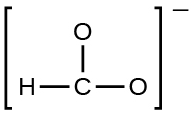# Problem: Write resonance forms that describe the distribution of electrons in each of these molecules or ions. the formate ion:

###### FREE Expert Solution

We are being asked to identify the resonance forms that describe the distribution of electrons in formate ion (HCO2-).

We will have to draw the Lewis Structure of HCO2- first.

Step 1: Determine the central atom in this molecule.

C and H are less electronegative than O but H can only make one bond
•  C is the central atom and is bonded to the H and 2 O atoms

Step 2: Calculate the total number of valence electrons present.

HCOO-:

Group              Valence Electrons

C          4A                    4 valence e x 1
H           1A                      1 valence e x 1
O           6A                      6 valence e- x 2
= 17 valence e

-1 charge                     +1 e­-

Total = 18 valence e-

Step 3: Draw the Lewis Structure for the molecule

O and C → prefers to have complete octet (8 e- around them)

90% (451 ratings)###### Problem Details

Write resonance forms that describe the distribution of electrons in each of these molecules or ions.

the formate ion: